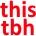R
Reactiflux

Script – 22-55 Feb 1

# Script – 22-55 Feb 1

SScript2/1/2022
I'm trying to run the function till `b.length === 1` and then return b What am I doing wrong? it returns undefined
``function superDigit(n, k) {  if (k === 1) return n;  n = n.toString();  let b = 0;  for (let i = 0; i < k; i++) {    n = n.concat(n);  }  for (let i in n) {    b += parseInt(n[i]);  }  function recall(bb) {    bb = bb.toString();    bbb = 0;    for (let i in bb) {      bbb += parseInt(bb[i]);    }    b = bbb;    if (b.length === 1) {      return b;    }  } recall(b);}``
``function superDigit(n, k) {  if (k === 1) return n;  n = n.toString();  let b = 0;  for (let i = 0; i < k; i++) {    n = n.concat(n);  }  for (let i in n) {    b += parseInt(n[i]);  }  function recall(bb) {    bb = bb.toString();    bbb = 0;    for (let i in bb) {      bbb += parseInt(bb[i]);    }    b = bbb;    if (b.length === 1) {      return b;    }  } recall(b);}``
SScriptyChris2/1/2022
because you don't return `recall(b)` call?
Gghardin1372/1/2022
wow you could probably stand to name your variables a bit better 🙂
SScript2/1/2022
``function recall(bb) {    bb = bb.toString();    bbb = 0;    for (let i in bb) {      bbb += parseInt(bb[i]);    }    b = bbb;    console.log("2", b);    return b;  }  if (b.length === 1) {    return b;  } else return recall(b);``
``function recall(bb) {    bb = bb.toString();    bbb = 0;    for (let i in bb) {      bbb += parseInt(bb[i]);    }    b = bbb;    console.log("2", b);    return b;  }  if (b.length === 1) {    return b;  } else return recall(b);``
LikeSScriptyChris2/1/2022
i meant thisSScript2/1/2022
😂 I was just rushing and giving it similar var names I tried that but still got undefined
SScriptyChris2/1/2022
is `b.length === 1`condition ever met?
SScript2/1/2022
yes
SScriptyChris2/1/2022
so `recall` at that case should return non-undefined `b` variable, because it surely is array/string with length property equal to 1
SScript2/1/2022
I'll look at my codeSScriptyChris2/1/2022
to be clear, you mean that `superDigit` returns undefined or `recall`? and are you sure that recursion ends sometime, not throws call stack exceeded error?
SS3BAS2/1/2022
Return the last line `return recall(b)` @Script
SScript2/1/2022
Okay so I am finally exceeding the call stack
``function superDigit(n, k) {  //   if (k === 1) return n;  n = n.toString();  let n2 = n;  let b = 0;  for (let i = 0; i < k - 1; i++) {    n = n.concat(n2);  }  for (let i in n) {    b += parseInt(n[i]);  }  function recall(newVal) {    newVal = newVal.toString();    point0 = 0;    for (let i in newVal) {      point0 += parseInt(newVal[i]);    }    b = point0;    if (b.length == 1) {      return b;    } else {      recall(b);    }  }  return recall(b);}``
``function superDigit(n, k) {  //   if (k === 1) return n;  n = n.toString();  let n2 = n;  let b = 0;  for (let i = 0; i < k - 1; i++) {    n = n.concat(n2);  }  for (let i in n) {    b += parseInt(n[i]);  }  function recall(newVal) {    newVal = newVal.toString();    point0 = 0;    for (let i in newVal) {      point0 += parseInt(newVal[i]);    }    b = point0;    if (b.length == 1) {      return b;    } else {      recall(b);    }  }  return recall(b);}``
How do I manage this
SS3BAS2/1/2022
Yeah then your base condition is never being met
SScript2/1/2022
It is actually b becomes 3 that's length of 1
SS3BAS2/1/2022
If you would properly hit the base condition you wouldn’t exceed the call stack Are you calling length on a number? Does that give you the amount of digits?
SScript2/1/2022
yes b is an integer
SS3BAS2/1/2022
What does the length of 0 return `0.length`
SScript2/1/2022SS3BAS2/1/2022
And `3.length`? I’m on phone so I can’t verify myself
UUUnknown User2/1/2022
Message Not Public
SS3BAS2/1/2022
Are you perhaps assuming that you can call `length` on a number without actually trying it out?
UUUnknown User2/1/2022
Message Not Public
SS3BAS2/1/2022
Yeah
SScript2/1/2022
same thing invalid
SS3BAS2/1/2022
So why are you calling `length` on a number 😛 Turn it to a string first
SScript2/1/2022my bad
SS3BAS2/1/2022
Don’t just assume code will work like you expect it to, you need to test and confirm even the smallest things heh Yeah all good
SScript2/1/2022
ayee still gettin undefined``function superDigit(n, k) {  n = n.toString();  let n2 = n;  let b = 0;  for (let i = 0; i < k - 1; i++) {    n = n.concat(n2);  }  for (let i in n) {    b += parseInt(n[i]);  }  function recall(newVal) {    newVal = newVal.toString();    point0 = 0;    for (let i in newVal) {      point0 += parseInt(newVal[i]);    }    b = point0;    if (b.toString().length === 1) {      return b;    } else {      recall(b);    }  }  return recall(b);}``
``function superDigit(n, k) {  n = n.toString();  let n2 = n;  let b = 0;  for (let i = 0; i < k - 1; i++) {    n = n.concat(n2);  }  for (let i in n) {    b += parseInt(n[i]);  }  function recall(newVal) {    newVal = newVal.toString();    point0 = 0;    for (let i in newVal) {      point0 += parseInt(newVal[i]);    }    b = point0;    if (b.toString().length === 1) {      return b;    } else {      recall(b);    }  }  return recall(b);}``
wait am I supposed to return `recall(b)` in recall()? Because I did now and it worked
SS3BAS2/1/2022
Yeah you are indeed Since it will evaluate to a number, but that number needs to be returned to the caller
UUUnknown User2/2/2022
2 Messages Not Public